/D3.js 4

# d3-voronoi

This module implements Steven J. Fortune’s algorithm for computing the Voronoi diagram or Delaunay triangulation of a set of two-dimensional points. This implementation is largely based on work by Raymond Hill.

Voronoi diagrams are not only visually attractive but practical tools for interaction, such as to increase the target area of points in a scatterplot. See “Strikeouts on the Rise” in The New York Times and this multi-line chart for examples; also see Tovi Grossman’s paper on bubble cursors for a related technique. Voronoi diagrams can also be used to automate label positioning, and Delaunay meshes are useful in computing adjacency or grouping of visual elements.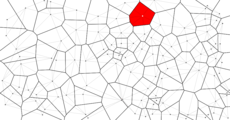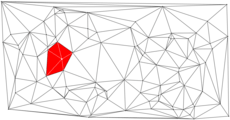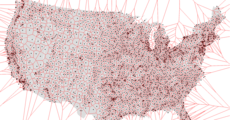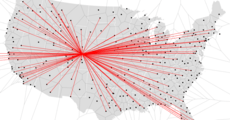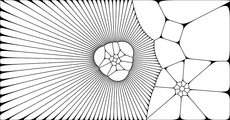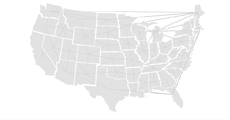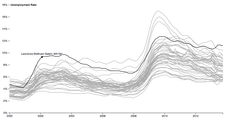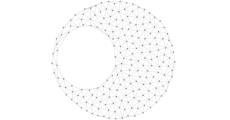## Installing

If you use NPM, `npm install d3-voronoi`. Otherwise, download the latest release. You can also load directly from d3js.org, either as a standalone library or as part of D3 4.0. AMD, CommonJS, and vanilla environments are supported. In vanilla, a `d3` global is exported:

```<script src="https://d3js.org/d3-voronoi.v1.min.js"></script>
<script>

var voronoi = d3.voronoi();

</script>```

Try d3-voronoi in your browser.

## API Reference

###### d3.voronoi() Source

Creates a new Voronoi layout with default x- and y- accessors and a null extent.

###### voronoi(data) Source

Computes the Voronoi diagram for the specified data points.

###### voronoi.x([x]) Source

If x is specified, sets the x-coordinate accessor. If x is not specified, returns the current x-coordinate accessor, which defaults to:

```function x(d) {
return d;
}```
###### voronoi.y([y]) Source

If y is specified, sets the y-coordinate accessor. If y is not specified, returns the current y-coordinate accessor, which defaults to:

```function y(d) {
return d;
}```
###### voronoi.extent([extent]) Source

If extent is specified, sets the clip extent of the Voronoi layout to the specified bounds and returns the layout. The extent bounds are specified as an array [[x0, y0], [x1, y1]], where x0 is the left side of the extent, y0 is the top, x1 is the right and y1 is the bottom. If extent is not specified, returns the current clip extent which defaults to null. A clip extent is required when using voronoi.polygons.

###### voronoi.size([size]) Source

An alias for voronoi.extent where the minimum x and y of the extent are ⟨0,0⟩. Equivalent to:

`voronoi.extent([[0, 0], size]);`
###### voronoi.polygons(data) Source

Returns a sparse array of polygons, one for each unique input point in the specified data points, corresponding to the cells in the computed Voronoi diagram. Equivalent to:

`voronoi(data).polygons();`

See diagram.polygons for more detail. Note: an extent is required.

###### voronoi.triangles(data) Source

Returns the Delaunay triangulation of the specified data array as an array of triangles. Each triangle is a three-element array of elements from data. Equivalent to:

`voronoi(data).triangles();`

See diagram.triangles for more detail.

Returns the Delaunay triangulation of the specified data array as an array of links. Each link has `source` and `target` attributes referring to elements in data. Equivalent to:

`voronoi(data).links();`

See diagram.links for more detail.

### Voronoi Diagrams

###### diagramSource

The computed Voronoi diagram returned by voronoi has the following properties:

• `edges` - an array of edges.
• `cells` - a sparse array of cells, one for each unique input point.

For each set of coincident input points, one of the points is chosen arbitrarily and assigned the associated cell; the other coincident input points’ entries are missing from the returned sparse array.

###### diagram.polygons() Source

Returns a sparse array of polygons clipped to the extent, one for each cell (each unique input point) in the diagram. Each polygon is represented as an array of points [x, y] where x and y are the point coordinates, and a `data` field that refers to the corresponding element in data. Polygons are open: they do not contain a closing point that duplicates the first point; a triangle, for example, is an array of three points. Polygons are also counterclockwise, assuming the origin ⟨0,0⟩ is in the top-left corner.

For each set of coincident input points, one of the points is chosen arbitrarily and assigned the associated polygon; the other coincident input points’ entries are missing from the returned sparse array.

###### diagram.triangles() Source

Returns the Delaunay triangulation of the specified data array as an array of triangles. Each triangle is a three-element array of elements from data. Since the triangulation is computed as the dual of the Voronoi diagram, and the Voronoi diagram is clipped by the extent, a subset of the Delaunay triangulation is returned.

Returns the Delaunay triangulation of the specified data array as an array of links, one for each edge in the mesh. Each link has the following attributes:

• `source` - the source node, an element in data.
• `target` - the target node, an element in data.

Since the triangulation is computed as the dual of the Voronoi diagram, and the Voronoi diagram is clipped by the extent, a subset of the Delaunay links is returned.

###### diagram.find(x, y[, radius]) Source

Returns the nearest site to point [x, y]. If radius is specified, only sites within radius distance are considered.

See Philippe Rivière’s bl.ocks.org/1b7ddbcd71454d685d1259781968aefc for an example.

###### cell

Each cell in the diagram is an object with the following properties:

• `site` - the site of the cell’s associated input point.
• `halfedges` - an array of indexes into diagram.edges representing the cell’s polygon.
###### site

Each site in the diagram is an array [x, y] with two additional properties:

• `index` - the site’s index, corresponding to the associated input point.
• `data` - the input data corresponding to this site.
###### edge

Each edge in the diagram is an array [[x0, y0], [x1, y1]] with two additional properties:

• `left` - the site on the left side of the edge.
• `right` - the site on the right side of the edge; null for a clipped border edge.

© 2010–2017 Michael Bostock